# Climb in percentage

The height difference between points A and B is 475 m. Calculate the percentage of route climbing if the horizontal distance places A, B is 7.4 km.

p =  6.42 %

### Step-by-step explanation: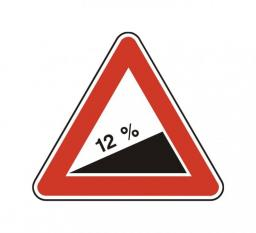Did you find an error or inaccuracy? Feel free to write us. Thank you!Tips to related online calculators
Check out our ratio calculator.
Do you want to convert length units?

#### You need to know the following knowledge to solve this word math problem:

We encourage you to watch this tutorial video on this math problem:

## Related math problems and questions:

• Railway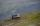Between points A, B, whose horizontal distance is 1.5 km railway line has 8promile climb. Between points B, C with horizontal distance of 900 m is climb 14promile. Calculate differences of altitudes between points A and C.
• Mountain railway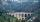Height difference between points A, B of railway line is 38.5 meters, their horizontal distance is 3.5 km. Determine average climb in permille up the track.
• RailwaysRailways climb 7.4 ‰. Calculate the height difference between two points on the railway distant 3539 meters.
• ClimbFor horizontal distance 4.2 km road rise by 6.3 m. Calculate the road pitch in ‰ (permille, parts per thousand).
• Altitude difference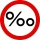What a climb in per mille of the hill long 4 km and the altitude difference is 6 meters?Between cities A and B is route 13 km long of stúpanie average 7‰. Calculate the height difference of cities A and B.
• Two municipalities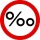The horizontal distance between municipalities is 39 km. Average sinking is 7 per mille. What is the difference in height between these municipalities?
• Cablecar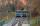Funicular on Petrin (Prague) was 408 meters long and overcomes the difference 106 meters in altitude. Calculate the angle of climb.
• Slope of trackCalculate the average slope (in permille and even in degrees) of the rail tracks between Prievidza (309 m AMSL) and Nitrianske Pravno (354 m AMSL), if the track is 11 km long.
• AMSL and skiingTomas skis from point A (3200m above sea level to place B. Hill has 20% descent. The horizontal distance between the start and finish is 2,5km. At what altitude is point B?
• RiverCalculate how many permille river Dunaj average falls, if on section long 957 km flowing water from 1454 m AMSL to 101 m AMSL.
• Horizontal distance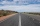The road has a gradient of 8%. How many meters will the road rise on a horizontal distance of 400m?
• The railway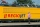The railway line has a gradient of 12 per mille. How many meters will it ascend to a horizontal distance of 4 km?
• Height differenceWhat height difference overcome if we pass road 1 km long with a pitch21 per mille?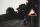A 5 km long road begins at an altitude 500 meters above sea level and ends at an altitude of 521 ASL. How many permille road rises?On the road sign, which informs the climb is 8.7%. The car drive 5 km along this road. What is the height difference that the car went to?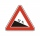I have a garden uphill, increasing from 0 to 4.5 m for a length of 25 m, how much is the climb in percent?